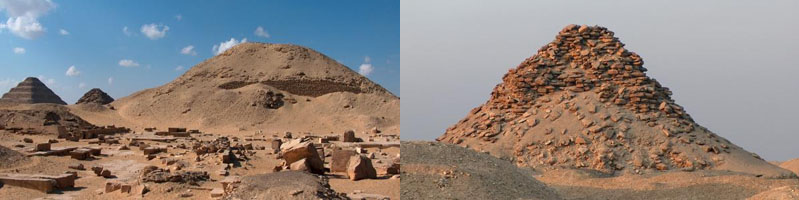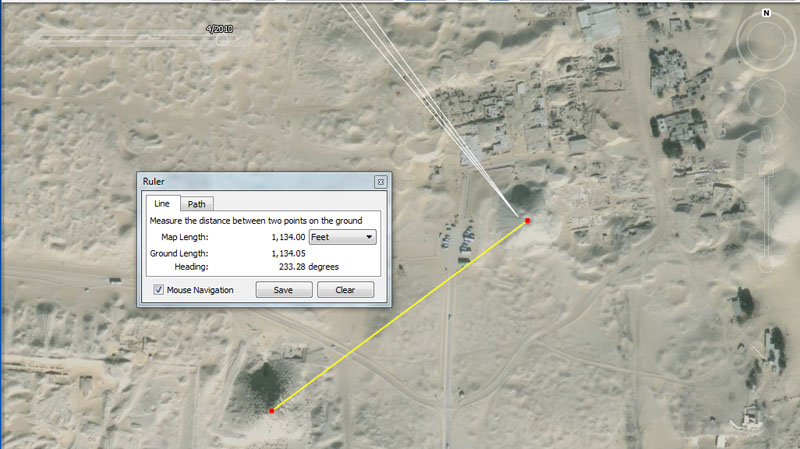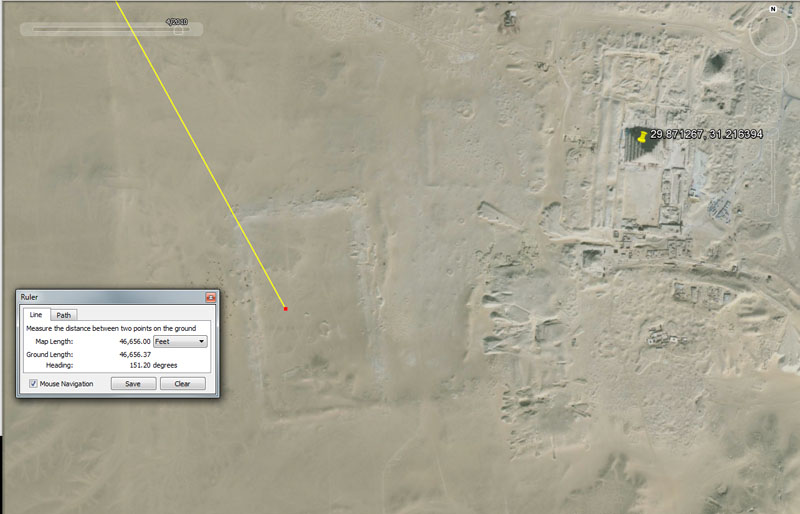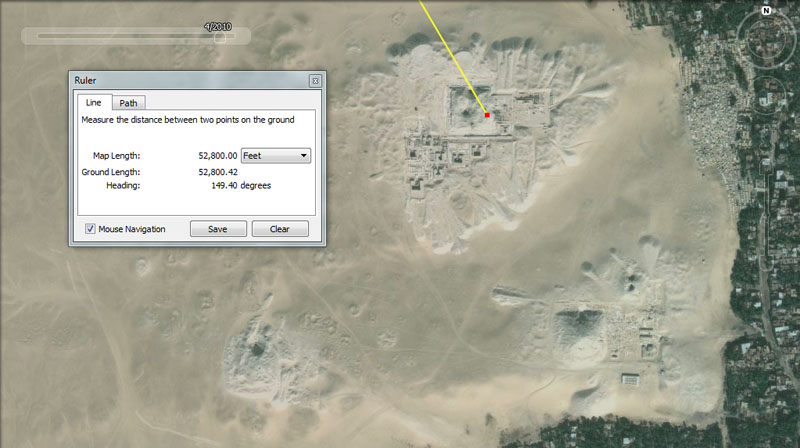THE PYRAMID OF TETI, NORTH SAQQARA.The very dilapidated pyramids of Teti (left) and Userkaf (right) at Northern Saqqara.3 lines run from the 3 large pyramids on the Giza Plateau and resolve on the Pyramid of Teti in North Saqqara. Another line (yellow) runs from the Pyramid of Userkaf for 1134' @ 233.28-degrees and resolves upon the Pyramid of Teti.

In this particular case, it's the Khafre Pyramid apex that provides the primary distance and angle code to the Pyramid of Teti. The distance is 46656' @ 142-degrees.

The distance of 46656' is 9.6 so-called Roman miles.

The angle value has no significance as a number in a mathematical progression, but sits at a primary, whole degree point of the 360-degree compass, which makes it very useful in navigation. However, the very close proximity number of 141.75-degrees would have great significance in a lunar cycle progression.

The apparent distance between the very wrecked Pyramid of Teti and its equally dilapidated neighbour, the Pyramid of Userkaf, could have been intended to convey 1134' @ 233.28-degrees return.

The Greek historian, Herodotus, was told by Egyptian priests that the Great Pyramid (756' actual) was 800' long. That particular "foot", referred to by the priests, was 11.34", which provides a very strong lunar code in a mathematical progression. Similarly the Khafre Pyramid (708.75' or precisely 15/16ths of the length of the Great Pyramid) equates to 750 X 11.34".

This increment crops up on megalithic observatory sites all over the world and at Stonehenge the intended size of the station stones rectangle was 264 feet long (1/20th of a mile) & 113.4 feet wide. Likewise, the Great Pyramid's base length = 2268' for 3-sides (1134' X 2).

In this case, the degree angle between Userkaf and Teti is 233.28-degrees, which value, in a mathematical progression achieves 46656, the same value (in feet) as the distance from Khafre Pyramid at Giza to these two pyramids at North Saqqara.A line runs from the Djoser Pyramid to the Pyramid of Seti for 2178' @ 226.8-degrees.

Because of the very wrecked condition of these outliers, one cannot verify that these close estimations were, precisely, the intended codes of distance and angle built into these positions. The design distance of separation could have been 2200'.

However, a distance of 2178' would be equally significant, as it would relate to the equatorial size of the Earth in a mathematical progression. The sum of 130680000' (24750 English miles) equates to 2178 X 60000.

At the same time, the intended angle appears to be 226.8-degrees (113.4 X 2).

Alternatively, the design distance from the Djoser Pyramid to the Userkaf Pyramid appears to have been 1134' @ 220-degrees. A coded distance of 1100' is also very possible.The very ancient, Great Enclosure at Saqqara sits 46656' @ 151.2 degrees away from the Great Pyramid's altar floor.

This distance is 9.6 so-called Roman miles (of 4860' each). Again the Romans, whose civilisation followed that of the Greeks, adopted much older measurement standards from the Egyptians and others. Everyone was using the whole parcel of numbers and measurement increments, although we, in modern times, used only small parts of the overall, original, systems nation-by-nation.

The Great Enclosure's dimensions will undoubtedly prove to hold codes of distance and angle. The eastern wall seems to lie on an angle of 171.875-degrees, which is strong lunar coding,

Its official measurements are 600 metres X 300 metres. The length of 600 metres translates fluidly to 1968.75'.

This is a very strong lunar code to do with the lunar year of 354.375-days and 1/18th of that period would be 19.6875-days (19 & 11/16ths) or 472.5 hours (remember, the height of the Khafre Pyramid was 472.5')

It's also very significant that, under the ancient Egyptian gold standard, the grain-weight of a beqa of gold was 196.875 grains. This same standard was adopted by the Romans for their Semuncia weight and by the Greeks for their Commercial Didrachma or Tridrachm weight.

The Great Enclosure (also called Gisr el-Mudir' or "wall of the director", would have functioned admirably as a space in which to work out logarithms, using a ground-etched 3,4,5 triangle of immense proportions.

The Roman foot could be read as both 11.664" (11 & 83/125ths) & 11.66666" (11 & 2/3rds), depending on the calculation being undertaken and this length to the Great Enclosure is 48000 Roman feet or 9600 Roman Paces of 58.32".

This distance to the Great Enclosure is 1/2800th of the 24883.2 Greek mile equatorial circumference.

The angle is also navigational coding and the sum of 151.2' would be 1/80th of 1-minute of equatorial arc. The Great Pyramid measured 1512' for two sides.

Alternatively, the distance to the Djoser Pyramid from Khafre Pyramid was 46656', and this holds true for adjacent, smaller pyramids to the ENE.

SOUTH SAQQARA.

PYRAMID OF PEPIA line runs for 52800' @ 149.5-degrees, from the Great Pyramid's surveying platform to the Pyramid of Pepi.

To the bottom left are the ruins of Merenre Pyramid, with the more complete Pyramid of Djedkare to the left. The small pyramid of an unidentified queen sits just northeast of Djedkare.

The distance from the Great Pyramid to the Pyramid of Pepi is, of course, 10 English miles.

With the very substantial Djoser Pyramid just to the North establishing the primary fix-point benchmark for the cluster, by both a coded distance and angle away from the Great Pyramid, these third station pyramids often carry no recognisable angle codes in their positions. The angle of 149.5-degrees has no significance.

The Pyramid of Pepi sits 52500' from Khafre Pyramid @ 147.75-degrees. This distance is, of course, 10 Greek miles.

The degree angle would have great significance as 147.65625 (147 & 21/32nds) as a very strong lunar code.

The Pyramid of Pepi sits 51840' from the Menkaure Pyramid @ 146.25-degrees.

The 5184 number is one of the most important and versatile to ancient navigational and astronomical calculations, for example:

1. The intended (coded) slope angle of the Great Pyramid was/is 51.84-degrees.

2. Each side of the Great Pyramid measures 72 Reeds of 10.5' each, which means the area the base occupies is 5184 square Reeds.

3. The Precession of the Equinoxes endures for 25920-years (72 X 360) or 5184-years X 5.

4. An English league of 16500' converts to a circumference of 51840', when multiplied by PI @ 3.141818182 (the form of PI used for "11" series numbers).

5. The 24883.2-mile circumference of the Earth equates to 518.4-miles X 48.

6. This distance between the Menkaure and Pepi pyramids is 1/2520th of the 24883.2-mile equatorial circumference.

The angle of 146.25-degrees from Menkaure to Pepi is without significance, however, an angle of 146.66666-degrees would be very significant. Likewise, a return angle of 326.7-degrees would be a part of an important mathematical progression related to the equatorial circumference of the Earth.

THE PYRAMID OF MERENRE.

This very dilapidated pyramid sits 53333.3333' @ 151.2-degrees from the Great Pyramid. Both the distance and the angle are code-bearing numbers.

The length is 1/3rd of 160000'. The ancient French had a foot of 10.66666" or 2 X 5.33333".

The sum of 2 sides of the Great Pyramid would equate to 1512' or 1/4th of 1-minute of equatorial arc.

From Khafre Pyramid Merenre the distance is, again, 52800' or 10 English miles @ 149.7-degrees.

The degree angle has no significance as a coded number.

From the Menkaure Pyramid to Merenre Pyramid the front side complies closely to 52000' (calendar coding), whereas the back complies closely to 52500'.

The degree angle to Merenre (148.2) is without significance, but would be very significant as a coded value @ 148.5-degrees.

PYRAMID OF DJEDKARE ISESI

From the Great Pyramid to the Djedkare Pyramid the distance is 54000' @149.6-degrees.

This distance would represent 1/2420th of the 24750 English mile equatorial circumference of the Earth.

The number 54 was much used in ancient calculations to do with lunar periods and the 6804-day lunar nutation cycle was 126 X 54-days (the Hebrew reed was 126" and the Greek foot was 12.6"). Both the lunar year (354.375-days) and the 7.2 lunar years (2551.5-days) monitored alongside 7-solar years in the lunisolar Sabbatical Calendar break into convenient divisions of 54).

The degree angle is without coded significance.

From Khafre Pyramid, Djedkare Pyramid stands off 53760' @148-degrees.

This distance id 1/2430th of the 24883.2 Greek mile equatorial circumference of the Earth. The Roman mile was 4860' or 5000 Roman feet of 11.664" (4860 is 2430 X 2).

The degree angle is without code-bearing significance.

From Menkaure Pyramid, Djedkare Pyramid stands off @ 53130' @ 146.5-degrees.

The Khafre Pyramid has a slope angle of 53.130-degrees, which is the degree angle generated on the hypotenuse of any 3,4,5 triangle. It seems that ancient architects wished to code this value into the landscape for permanent mnemonic reference.

The angle to Djedkara would have tremendous significance as 146.6666-degrees (navigation) or 326.7-degrees return (equatorial circumference).

Continue